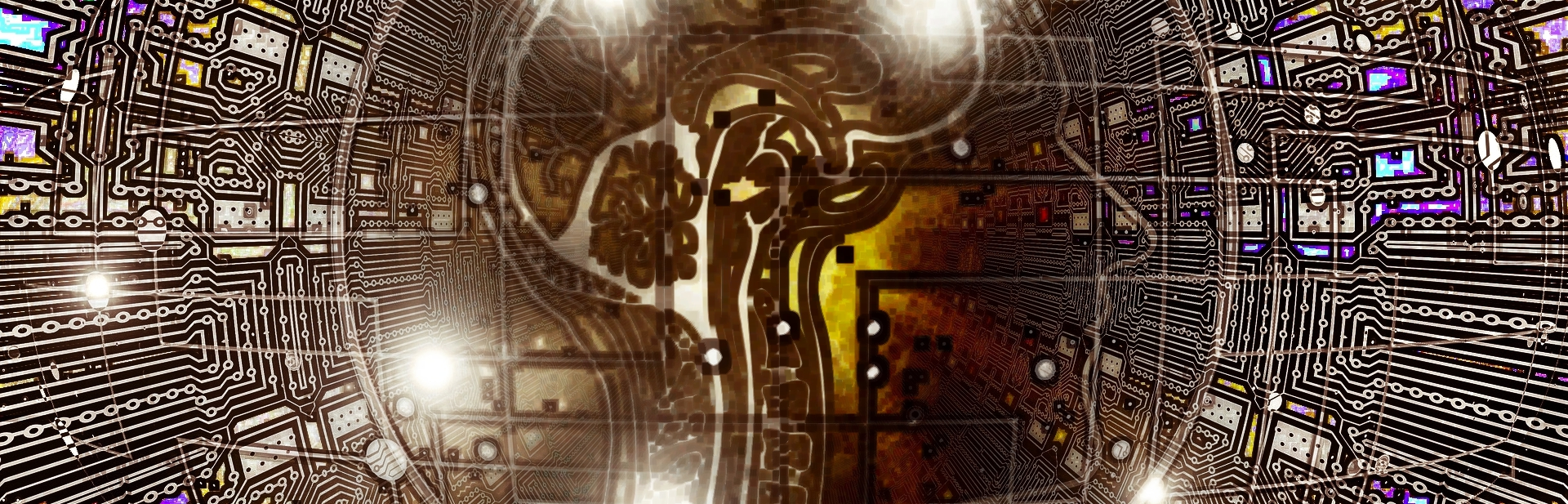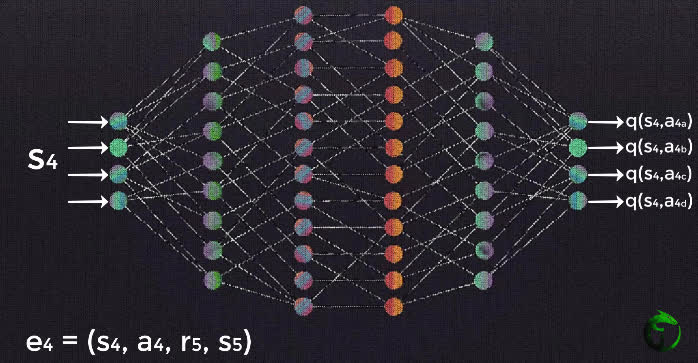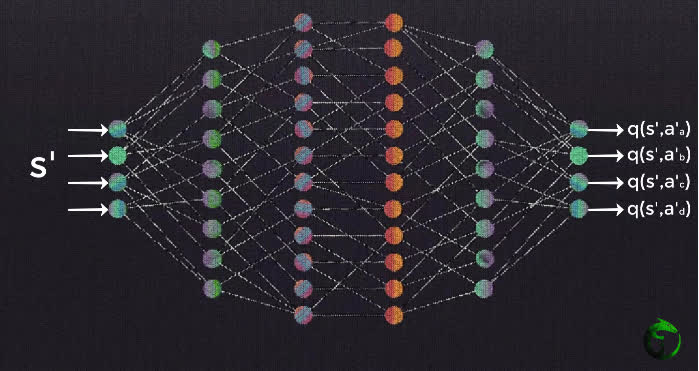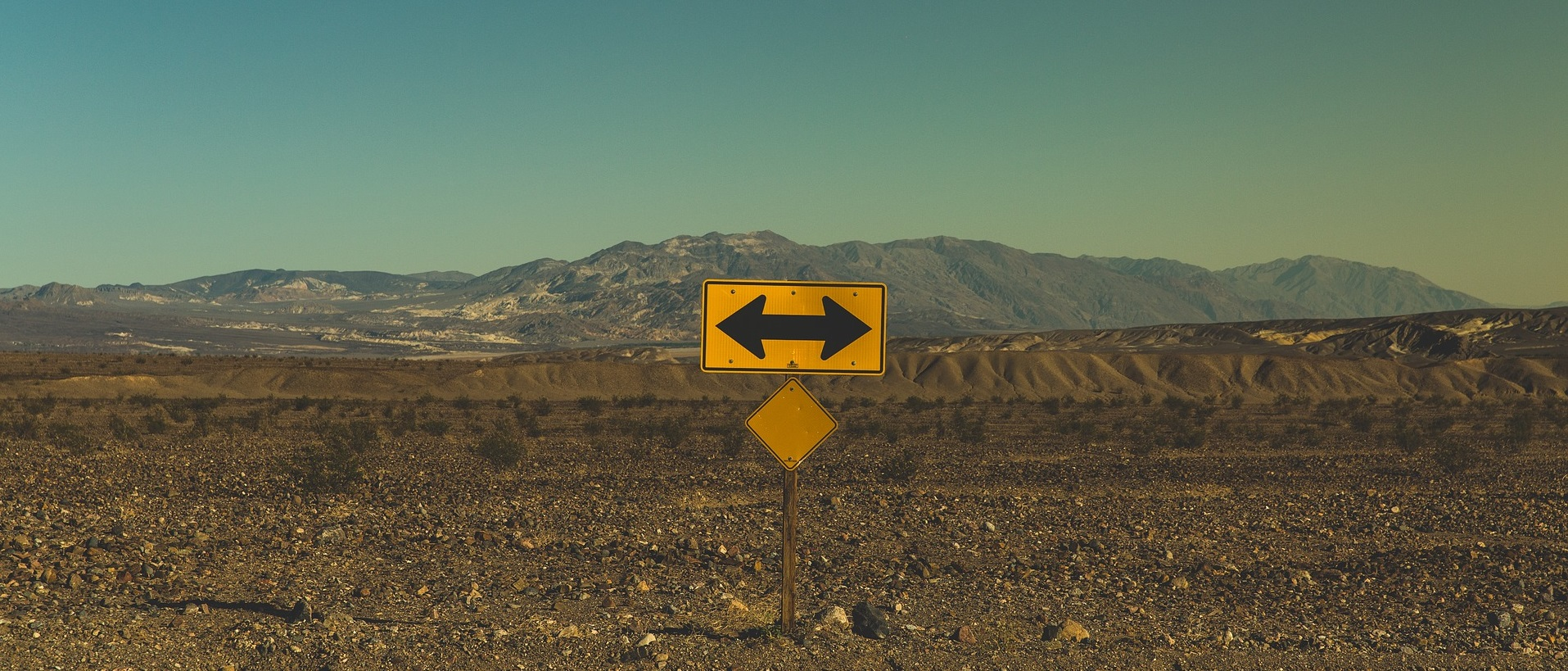# Reinforcement Learning - Goal Oriented Intelligence

Deep Learning Course 4 of 5 - Level: Advanced

## Training a Deep Q-Network - Reinforcement Learning

### video

expand_more chevron_left

### text

expand_more chevron_left

### Training a deep Q-network with replay memory

What's up, guys? In this post, we'll continue our discussion of deep Q-networks and focus in on the complete algorithmic details of the underlying training process. With this, we'll see exactly how the replay memory that was introduced in the previous post is utilized during training as well. So, let's get to it!What do we know so far about deep Q-learning? Well, we know about the deep Q-network architecture, and we also have been introduced to replay memory . We're now going to see exactly how the training process works for a DQN by utilizing this replay memory.

Here is a snapshot summary of what all we've went over before we ended last time.

1. Initialize replay memory capacity.
2. Initialize the network with random weights.
3. For each episode:
1. Initialize the starting state.
2. For each time step:
1. Select an action.
• Via exploration or exploitation
2. Execute selected action in an emulator.
3. Observe reward and next state.
4. Store experience in replay memory.

Make sure you've got an understanding of all this. All of these steps have occurred before the actual training of the neural network starts. At this point, we're inside of a single time step within a single episode. Now, we'll pick up right where we left off after the experience is stored in replay memory to discuss what exactly happens during training.

### The policy network

After storing an experience in replay memory, we then sample a random batch of experiences from replay memory. For ease of understanding, though, we're going to explain the remaining process for a single sample, and then you can generalize the idea to an entire batch.

Alright, so from a single experience sample from replay memory, we then preprocess the state (grayscale conversion, cropping, scaling, etc.), and pass the preprocessed state to the network as input. Going forward, we'll refer to this network as the policy network since its objective is to approximate the optimal policy by finding the optimal Q-function.The input state data then forward propagates through the network, using the same forward propagation technique that we've discussed for any other general neural network. The model then outputs an estimated Q-value for each possible action from the given input state.

The loss is then calculated. We do this by comparing the Q-value output from the network for the action in the experience tuple we sampled and the corresponding optimal Q-value, or target Q-value, for the same action.

Remember, the target Q-value is calculated using the expression from the right hand side of the Bellman equation. So, just as we saw when we initially learned about plain Q-learning earlier in this series, the loss is calculated by subtracting the Q-value for a given state-action pair from the optimal Q-value for the same state-action pair.

\begin{eqnarray*} q_{\ast }\left( s,a\right) - q(s,a)&=&loss \\E\left[ R_{t+1}+\gamma \max_{a^{\prime }}q_{\ast }\left( s^\prime,a^{\prime }\right)\right] - E\left[ \sum_{k=0}^{\infty }\gamma ^{k}R_{t+k+1}\right]&=&loss \end{eqnarray*}

### Calculating the $$\max$$ term

When we are calculating the optimal Q-value for any given state-action pair, notice from the equation for calculating loss that we used above, we have this term here that we must compute:

$$\max_{a^{\prime }}q_{\ast }\left( s^\prime,a^{\prime }\right)$$

Recall that $$s^\prime$$ and $$a^{\prime}$$ are the state and action that occur in the following time step. Previously, we were able to find this $$\max$$ term by peeking in the Q-table, remember? We'd just look to see which action gave us the highest Q-value for a given state.

Well that's old news now with deep Q-learning. In order to find this $$\max$$ term now, what we do is pass $$s^\prime$$ to the policy network, which will output the Q-values for each state-action pair using $$s^\prime$$ as the state and each of the possible next actions as $$a^\prime$$. Given this, we can obtain the $$\max$$ Q-value over all possible actions taken from $$s^\prime$$, giving us $$\max_{a^{\prime}}q_{*}(s^\prime,a^{\prime})$$.Once we find the value of this $$\max$$ term, we can then calculate this term for the original state input passed to the policy network.

\begin{eqnarray*} \\E\left[ R_{t+1}+\gamma \max_{a^{\prime }}q_{\ast }\left( s^\prime,a^{\prime }\right)\right] \end{eqnarray*}

Why do we need to calculate this term again?

Ah, yes, this term enables us to compute the loss between the Q-value given by the policy network for the state-action pair from our original experience tuple and the target optimal Q-value for this same state-action pair.

So, to quickly touch base, note that we first forward passed the state from our experience tuple to the network and got the Q-value for the action from our experience tuple as output. We then passed the next state contained in our experience tuple to the network to find the $$\max$$ Q-value among the next actions that can be taken from that state. This second step was done just to aid us in calculating the loss for our original state-action pair.

This may seem a bit odd, but let it sink in for a minute and see if the idea clicks.

### Training the policy network

Alright, so after we're able to calculate the optimal Q-value for our state-action pair, we can calculate the loss from our policy network between the optimal Q-value and the Q-value that was output from the network for this state-action pair.

Gradient descent is then performed to update the weights in the network in attempts to minimize the loss, just like we've seen in all other previous networks we've covered on this channel. In this case, minimizing the loss means that we're aiming to make the policy network output Q-values for each state-action pair that approximate the target Q-values given by the Bellman equation.Up to this point, everything we've gone over was all for one single time step. We then move on to the next time step in the episode and do this process again and again time after time until we reach the end of the episode. At that point, we start a new episode, and do that over and over again until we reach the max number of episodes we've set. We'll want to keep repeating this process until we've sufficiently minimized the loss.

### Wrapping up

Admittingly, between the last post and this one, that was quite a number of steps, so let's go over this summary to bring it all together.

1. Initialize replay memory capacity.
2. Initialize the network with random weights.
3. For each episode:
1. Initialize the starting state.
2. For each time step:
1. Select an action.
• Via exploration or exploitation
2. Execute selected action in an emulator.
3. Observe reward and next state.
4. Store experience in replay memory.
5. Sample random batch from replay memory.
6. Preprocess states from batch.
7. Pass batch of preprocessed states to policy network.
8. Calculate loss between output Q-values and target Q-values.
• Requires a second pass to the network for the next state
9. Gradient descent updates weights in the policy network to minimize loss.

Take some time to go over this algorithm and see if you now have the full picture for how policy networks, experience replay, and training all come together. Let me know your thoughts in the comments!In the next video, we'll see what kind of problems could be introduced by the process we covered here. Anyone want to try to guess? Given the problems that we'll discuss next time, we'll see how we can actually improve the training process by introducing a second network. Yes, two neural networks being used at the same time. Well kind of, we'll just have to wait and see. I'll see ya in the next one!

### quiz

expand_more chevron_leftDEEPLIZARD Message notifications

### resources

expand_more chevron_left
Welcome back to this series on reinforcement learning! In this video, we'll continue our discussion of deep Q-networks and focus in on how exactly a DQN is trained. With this, we'll see how replay memory is utilized during training. So, let's get to it! Sources: Reinforcement Learning: An Introduction, Second Edition by Richard S. Sutton and Andrew G. Bartow http://incompleteideas.net/book/RLbook2020.pdf Playing Atari with Deep Reinforcement Learning by Deep Mind Technologies https://www.cs.toronto.edu/~vmnih/docs/dqn.pdf Jürgen Schmidhuber TED Talk: https://youtu.be/T1Ogwa76yQo 🕒🦎 VIDEO SECTIONS 🦎🕒 00:00 Welcome to DEEPLIZARD - Go to deeplizard.com for learning resources 00:30 Help deeplizard add video timestamps - See example in the description 08:38 Collective Intelligence and the DEEPLIZARD HIVEMIND 💥🦎 DEEPLIZARD COMMUNITY RESOURCES 🦎💥 👋 Hey, we're Chris and Mandy, the creators of deeplizard! 👀 CHECK OUT OUR VLOG: 🔗 https://youtube.com/deeplizardvlog 👉 Check out the blog post and other resources for this video: 🔗 https://deeplizard.com/learn/video/0bt0SjbS3xc 💻 DOWNLOAD ACCESS TO CODE FILES 🤖 Available for members of the deeplizard hivemind: 🔗 https://deeplizard.com/resources 🧠 Support collective intelligence, join the deeplizard hivemind: 🔗 https://deeplizard.com/hivemind 🤜 Support collective intelligence, create a quiz question for this video: 🔗 https://deeplizard.com/create-quiz-question 🚀 Boost collective intelligence by sharing this video on social media! ❤️🦎 Special thanks to the following polymaths of the deeplizard hivemind: Tammy Prash Zach Wimpee 👀 Follow deeplizard: Our vlog: https://youtube.com/deeplizardvlog Facebook: https://facebook.com/deeplizard Instagram: https://instagram.com/deeplizard Twitter: https://twitter.com/deeplizard Patreon: https://patreon.com/deeplizard YouTube: https://youtube.com/deeplizard 🎓 Deep Learning with deeplizard: Fundamental Concepts - https://deeplizard.com/learn/video/gZmobeGL0Yg Beginner Code - https://deeplizard.com/learn/video/RznKVRTFkBY Intermediate Code - https://deeplizard.com/learn/video/v5cngxo4mIg Advanced Deep RL - https://deeplizard.com/learn/video/nyjbcRQ-uQ8 🎓 Other Courses: Data Science - https://deeplizard.com/learn/video/d11chG7Z-xk Trading - https://deeplizard.com/learn/video/ZpfCK_uHL9Y 🛒 Check out products deeplizard recommends on Amazon: 🔗 https://amazon.com/shop/deeplizard 📕 Get a FREE 30-day Audible trial and 2 FREE audio books using deeplizard's link: 🔗 https://amzn.to/2yoqWRn 🎵 deeplizard uses music by Kevin MacLeod 🔗 https://youtube.com/channel/UCSZXFhRIx6b0dFX3xS8L1yQ 🔗 http://incompetech.com/ ❤️ Please use the knowledge gained from deeplizard content for good, not evil.

expand_more chevron_leftDEEPLIZARD Message notifications